# Cartesian Coordinates

Cartesian Coordinates

John wants to meet his friend, Chris, who lives in another town. John starts from his house but does not know the address. Do you think it will be easy for John to reach his friend's house? Definitely, no! If John is given the right address, he can reach there easily. The same applies to a cartesian coordinate plane. If we want to locate a point on a cartesian coordinate plane, we must know the exact location of the point. The location of the point is known as its cartesian coordinates or coordinates of the point.Have you ever wondered about the uses of cartesian coordinates or the cartesian plane? They have several uses; for example, Google maps use the cartesian coordinate plane to show the locations.

In this short lesson, you will learn all about the cartesian coordinate plane. You will learn how to locate a point and plot a point in the different quadrants of a cartesian coordinate plane, and solve problems based on the cartesian coordinate system.

Let's begin!

## Lesson Plan

 1 What Is a Cartesian Coordinate Plane? 2 Solved Examples on Cartesian Coordinate 3 Interactive Questions on Cartesian Coordinates

## What Is a Cartesian Coordinate Plane?

A cartesian coordinate plane is a system of two perpendicular number lines on which we locate points.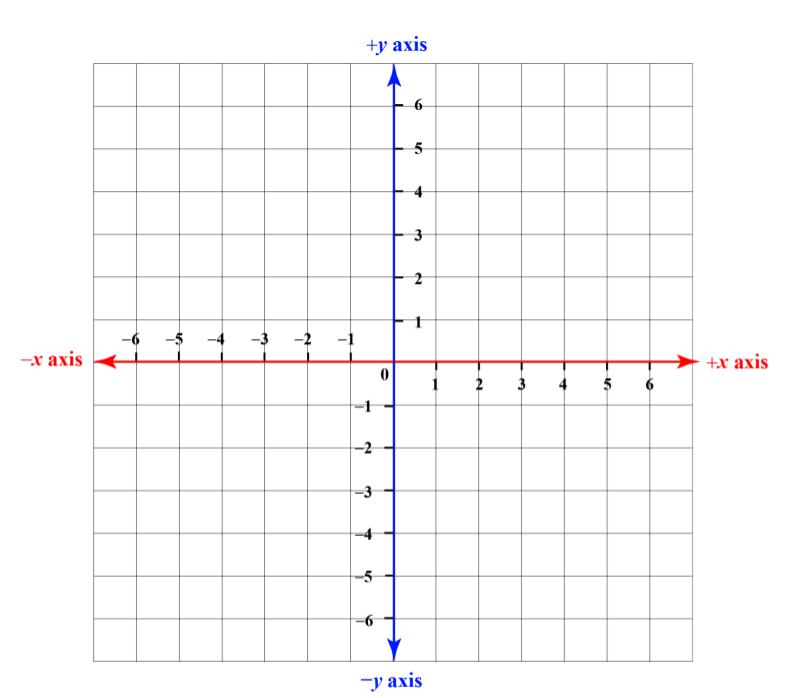The cartesian coordinate plane consists of two perpendicular lines, x-axis and y-axis.

The horizontal line is called the x-axis.

The vertical line is called the y-axis.

The intersection point of these two axes is called origin and is represented by $$O$$.

The axes split through the origin, which is marked as 0 on the graph.

The side on the right of 0 is positive, while everything on the left is negative.

Similarly, as you go higher, the numbers are positive, while the negative numbers are written below 0

The positive x-axis has positive numbers such as 1, 2, 3, 4,…..

The negative x-axis has negative numbers such as -1, -2, -3, -4,…..

The positive y-axis has positive numbers such as 1, 2, 3, 4,…..

The negative y-axis has positive numbers such as -1, -2, -3, -4,…..

A cartesian coordinate plane can simply be called a coordinate plane or a plane.

It represents a 2-dimensional (2D) plane.

The cartesian coordinate system is sometimes also referred as the cartesian coordinate graph.

## Cartesian Coordinates

A cartesian coordinate plane can have infinite points on it, but each point has its own unique coordinates.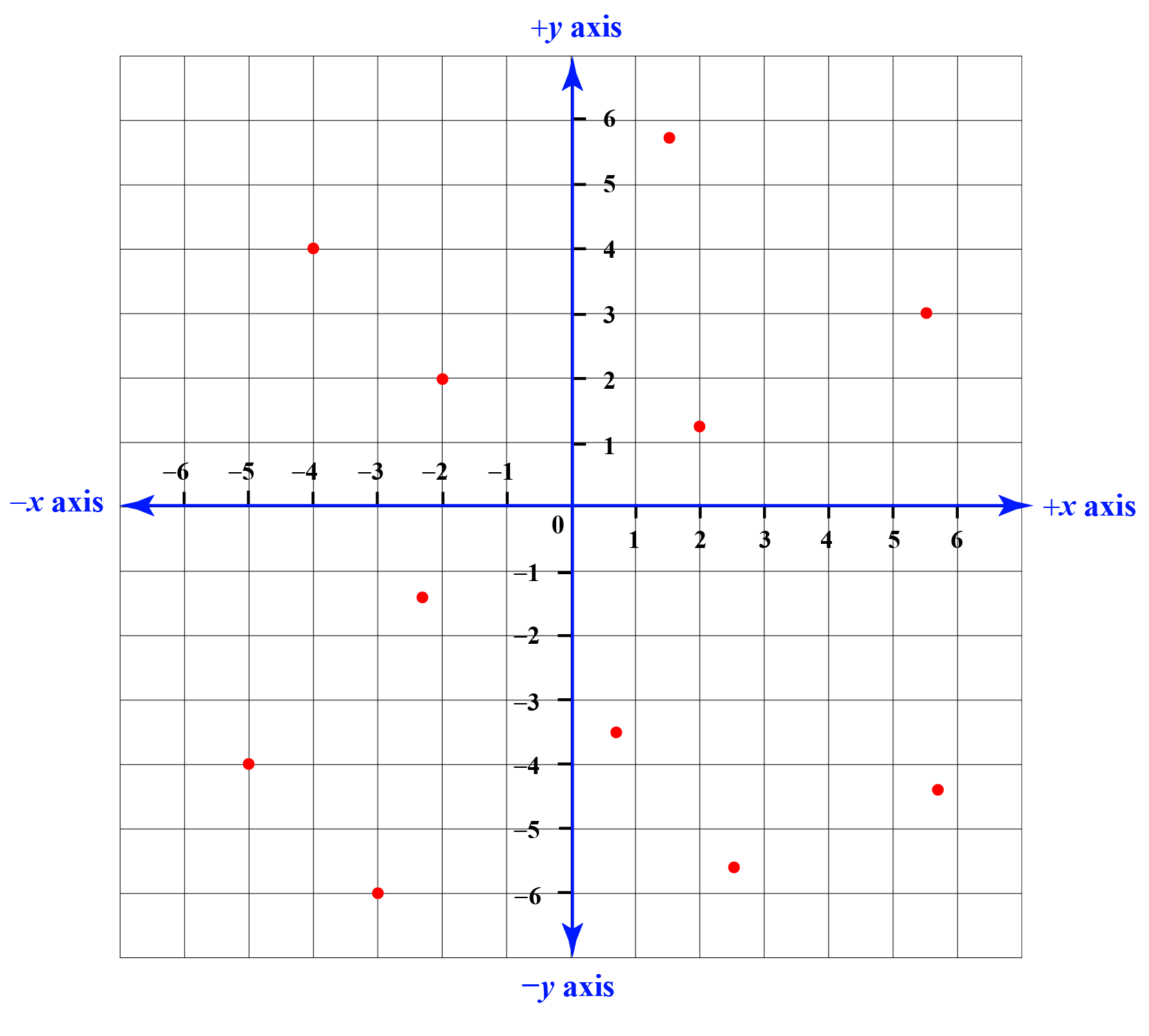The red dots in the cartesian plane above are the cartesian points, and each point has unique coordinates known as cartesian coordinates.

## Cartesian Coordinate on a Plane

How will you locate the coordinates of a point on a plane?

How will you find the coordinates by looking at a point?

We will find answers to these two questions in this section.

Consider a point $$P$$ on the coordinate plane.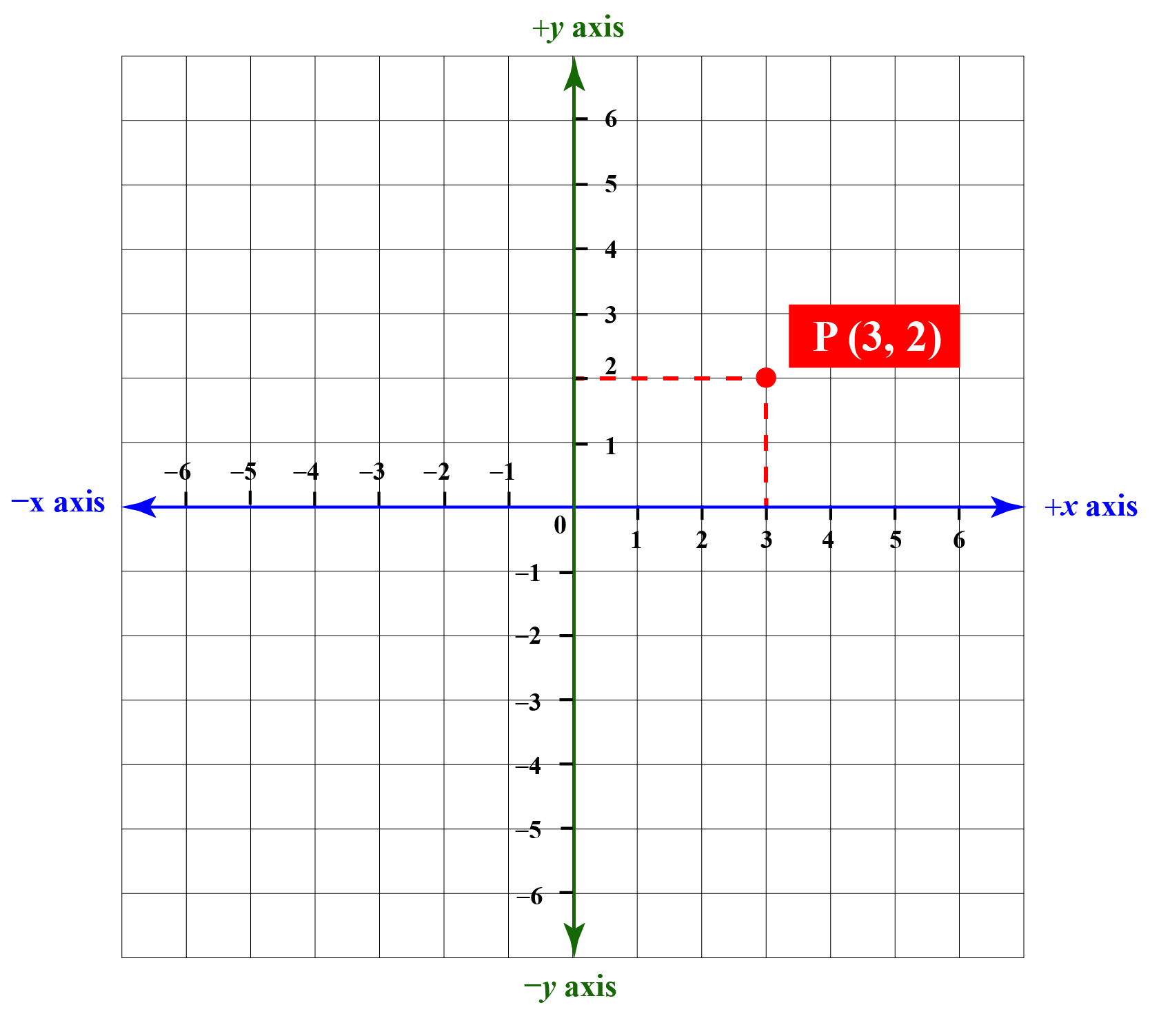There are two numbers written within the brackets.

This pair of numbers (3, 2) are known as the coordinates of the point $$P$$ or we can say that this is the location of the point $$P$$ on the cartesian plane.

The coordinates of point $$P$$ are: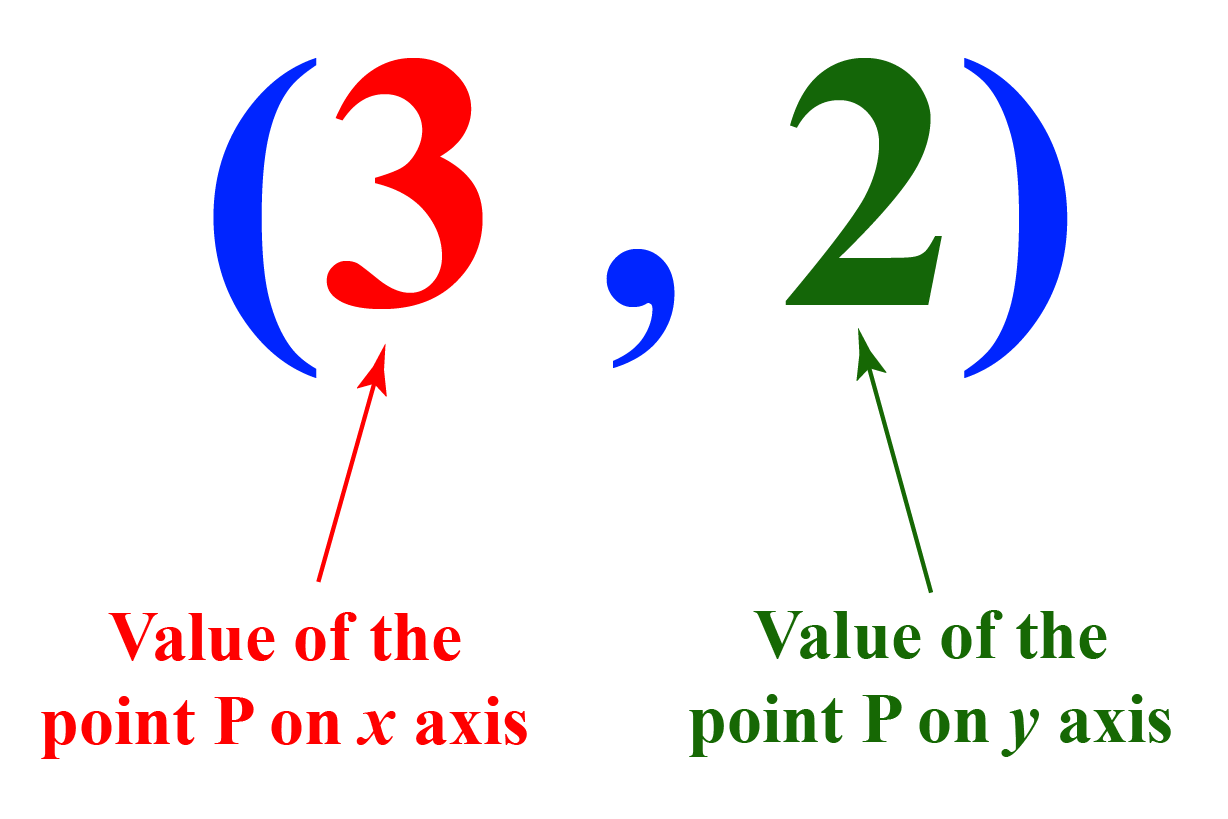This is how we write the coordinates of a point $$(x, y)$$ i.e. (value on the x-axis, the value on the y-axis).

Use the simulation given below to observe how the coordinates change when the point is moved across the graph.Tips and Tricks
1. Any point that lies on the x-axis has its y-coordinate zero.
2. Any point that lies on the y-axis has its x-coordinate zero.
3. For any line which is parallel to the y-axis, the x-coordinate of any point on the line always remains the same.
4. For any line which is parallel to the x-axis, the y-coordinate of any point on the line always remains the same.

## Coordinate Plane Quadrants on a 2-D Plane

The two axes x-axis and y-axis divide the graph into four parts.

These four parts are known as coordinate plane quadrants or we can simply call them quadrants.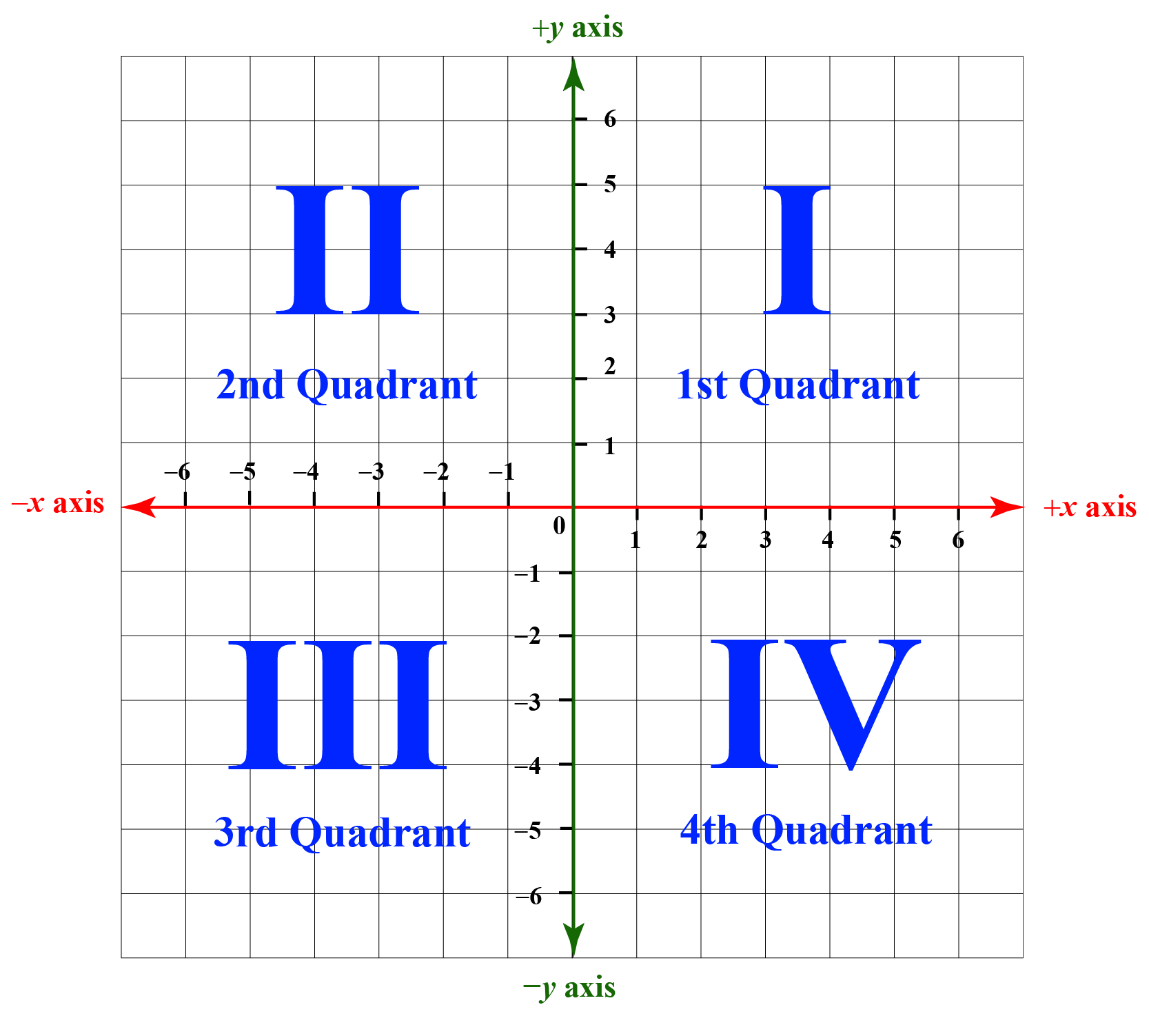## Coordinates of Points on Coordinate Plane Quadrants

If we divide the points into the four quadrants, then the sign of the points will vary according to the quadrant in which it lies.

For example, point (3, -2) lies in the 4th quadrant.

Let’s see how to identify this.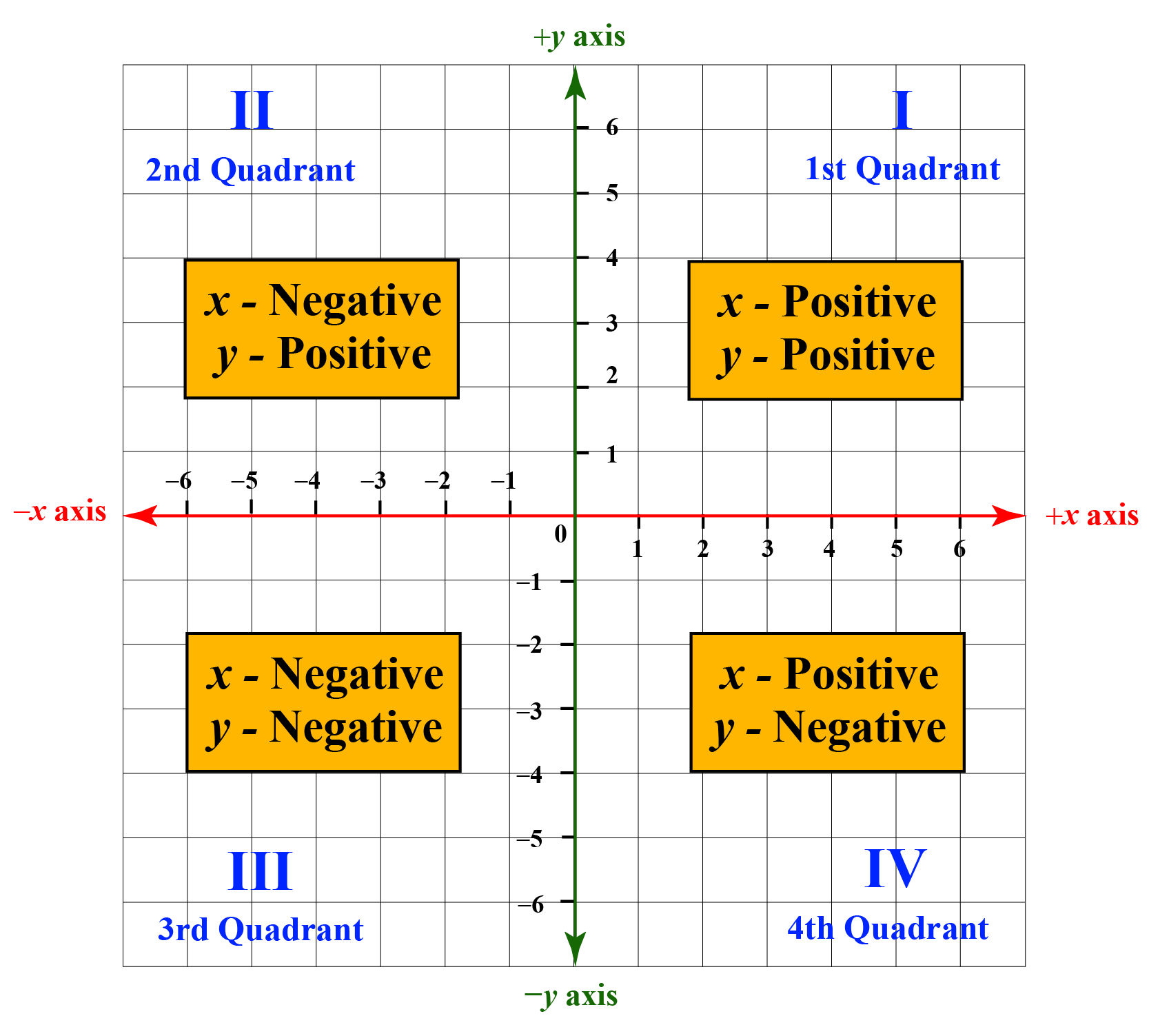Example 1

William wants to throw a ball into a bucket which is placed on the coordinates (3, 10).

If William is standing on the coordinates (3, 5), how far will he have to throw the ball?

Solution

To calculate how far William will have to throw the ball, we need to calculate the distance between William and the bucket.

The x-coordinate of William's location and the bucket is 3

Thus, they are on a line parallel to the y-axis.

Hence, the distance between William and the bucket can be calculated as the difference of the y-coordinates of both the points.

$10 - 5 = 5 units$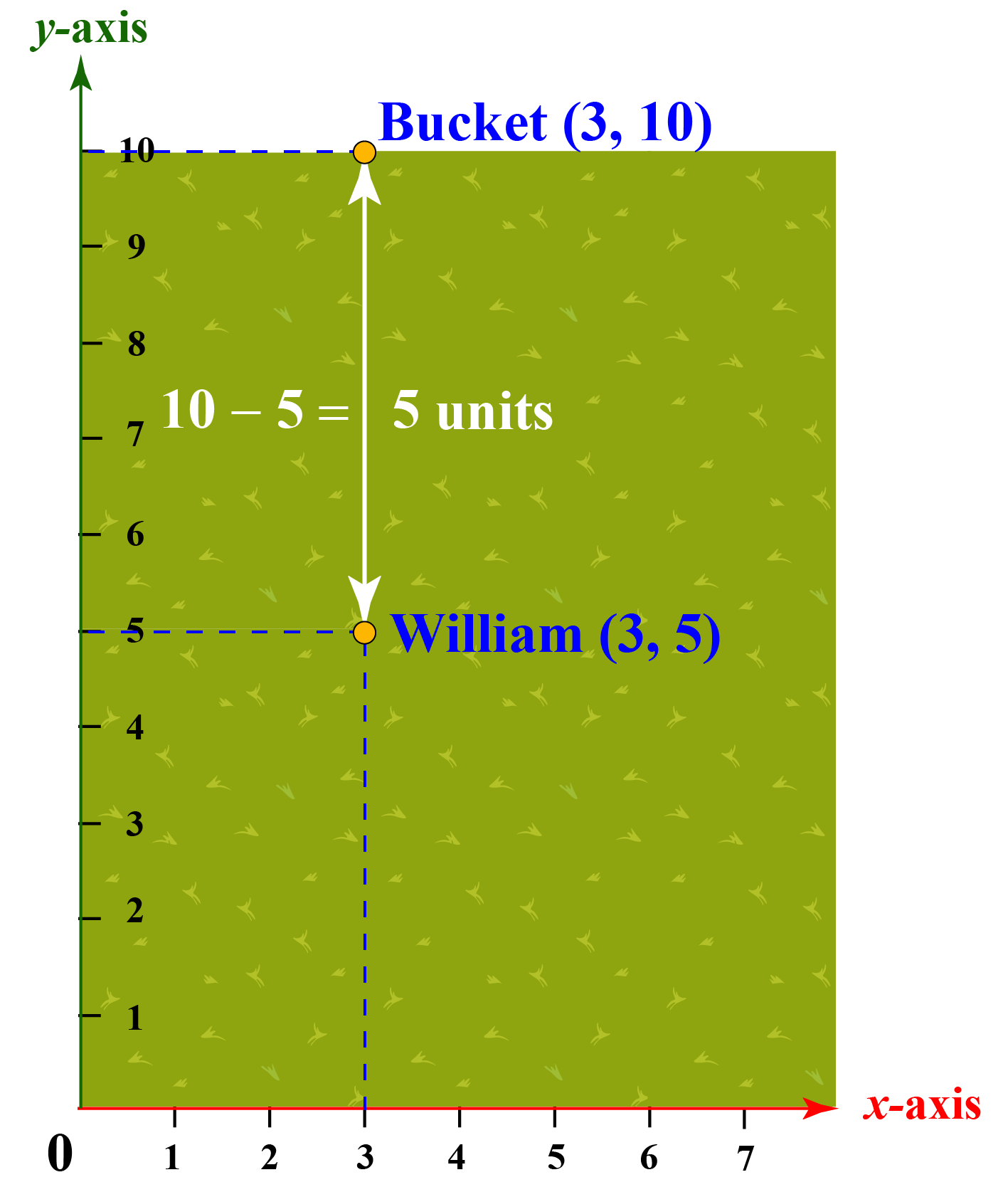Thus, William has to throw the ball $$5$$ units away
 Example 2

If point P $$(3, 4)$$ and point Q $$(5, 7-a)$$ lie on a line parallel to the x-axis, then what is the value of $$a$$?

Solution

We know that both the points P and Q lie on a line parallel to the x-axis, thus, their y-coordinates are the same.

\begin{align} 7 - a &= 4\\[0.2cm] a &= 7 - 4\\[0.2cm] a &= 3 \end{align}

 $$\therefore$$ $$a=3$$
 Example 3

Jacob and Ethan want to make a frame using the coordinates $$(1, 2), (3, 2), (3, 0), (1, 0)$$.

Based on the coordinates, Jacob says that the frame will be a square while Ethan says that the frame will be a parallelogram.

Can you identify who is right?

Solution

We need to draw the above coordinates on a cartesian plane to check the shape they will form.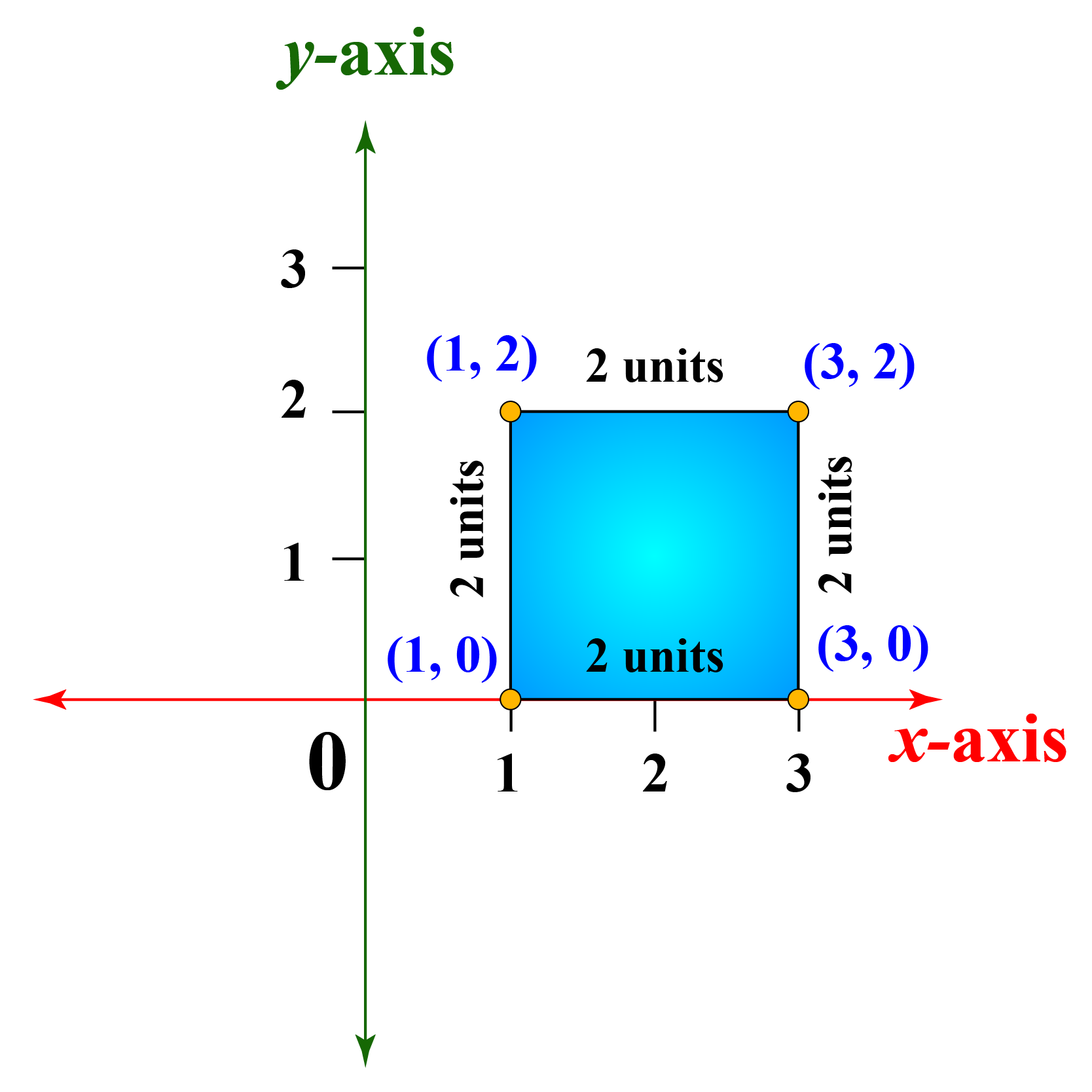We can clearly see that the figure thus obtained is a square as all the four sides are equal and all the four interior angles are $$90^{\circ}$$.

 $$\therefore$$ Jacob is right.
 Example 4

The cartesian coordinate plane below represents a city map with 5 different locations.

Find the cartesian coordinates for each of these locations.

A - School; B - Hospital; C - Cinema hall; D - Police department; E - Zoo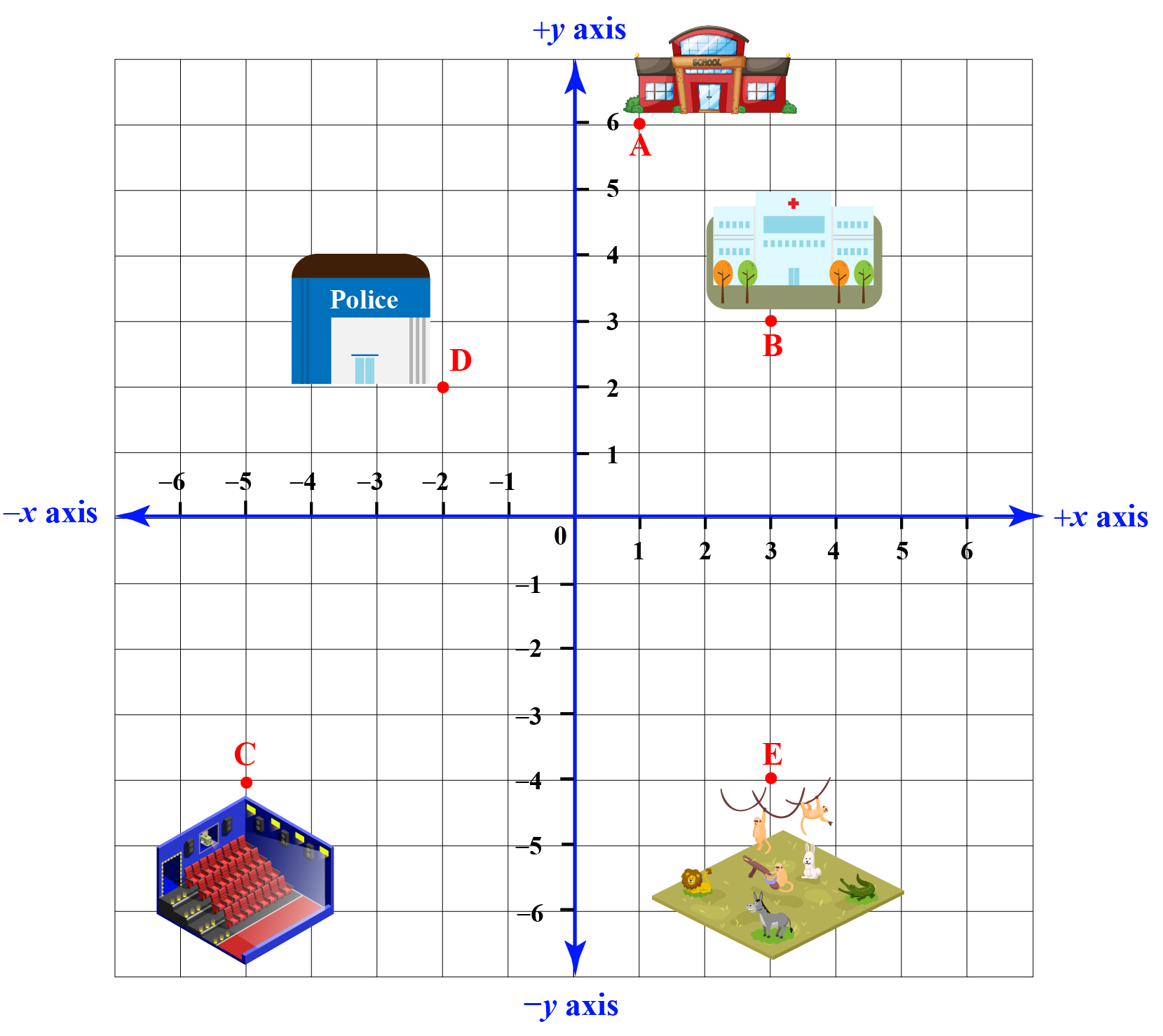Solution

 School is situated at (1, 6) Hospital is Situated at (3, 3) Cinema Hall is situated at (-5, -4) Police department is situated at (-2, 2) Zoo is situated at (3, -4)
 Example 5

If the four quadrants represent the following 4 states of the US:

Can you identify in which state these points lie?
A (4, -2)
B (-3, -5)
C (1, 2)
D (-7, 1)
E (-2, -6)

Solution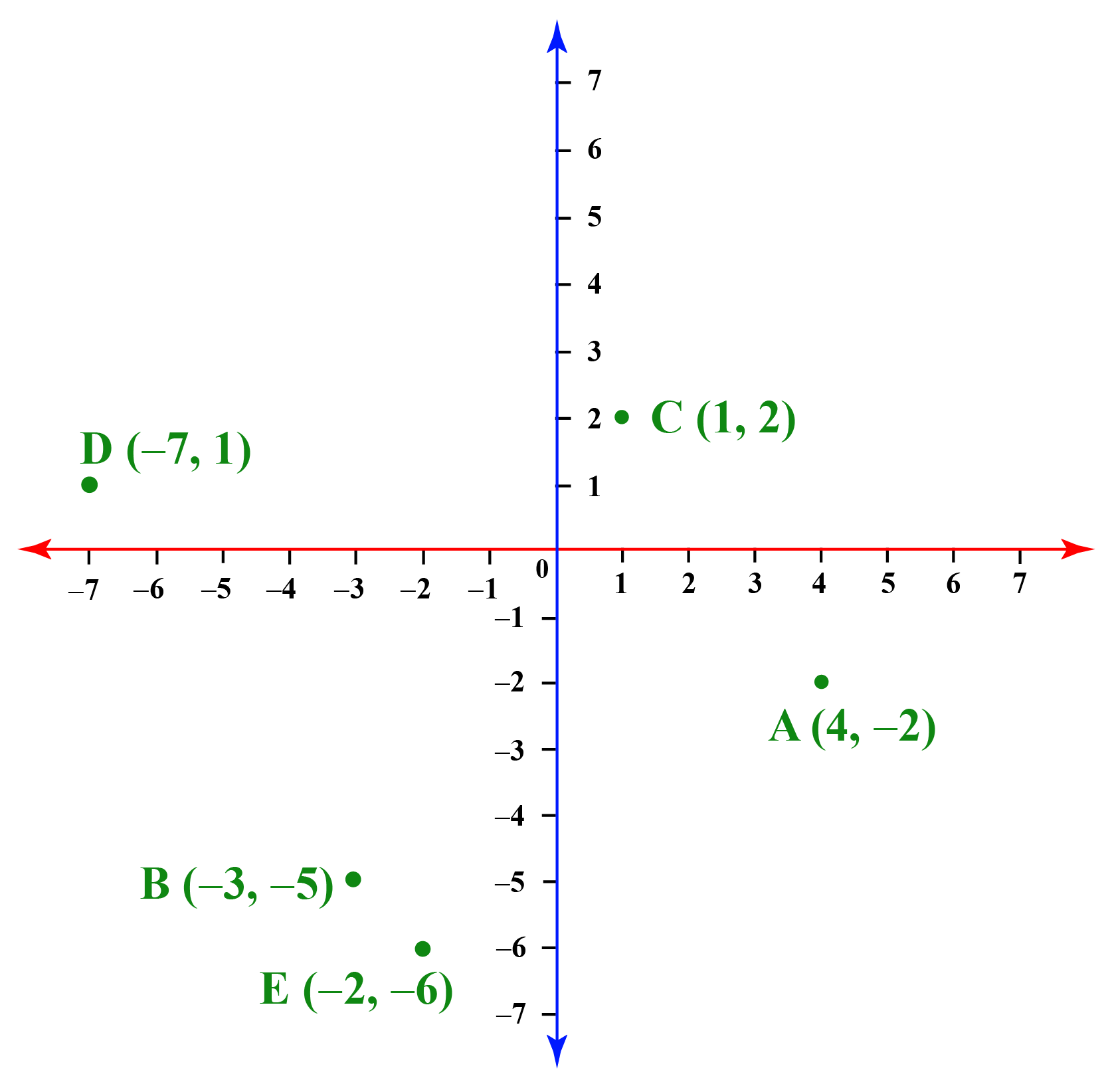Point A lies in Arizona Point B lies in Texas Point C lies in California Point D lies in Florida Point E lies in Texas
 Example 6

In which quadrants do the points P, Q, R and S lie in?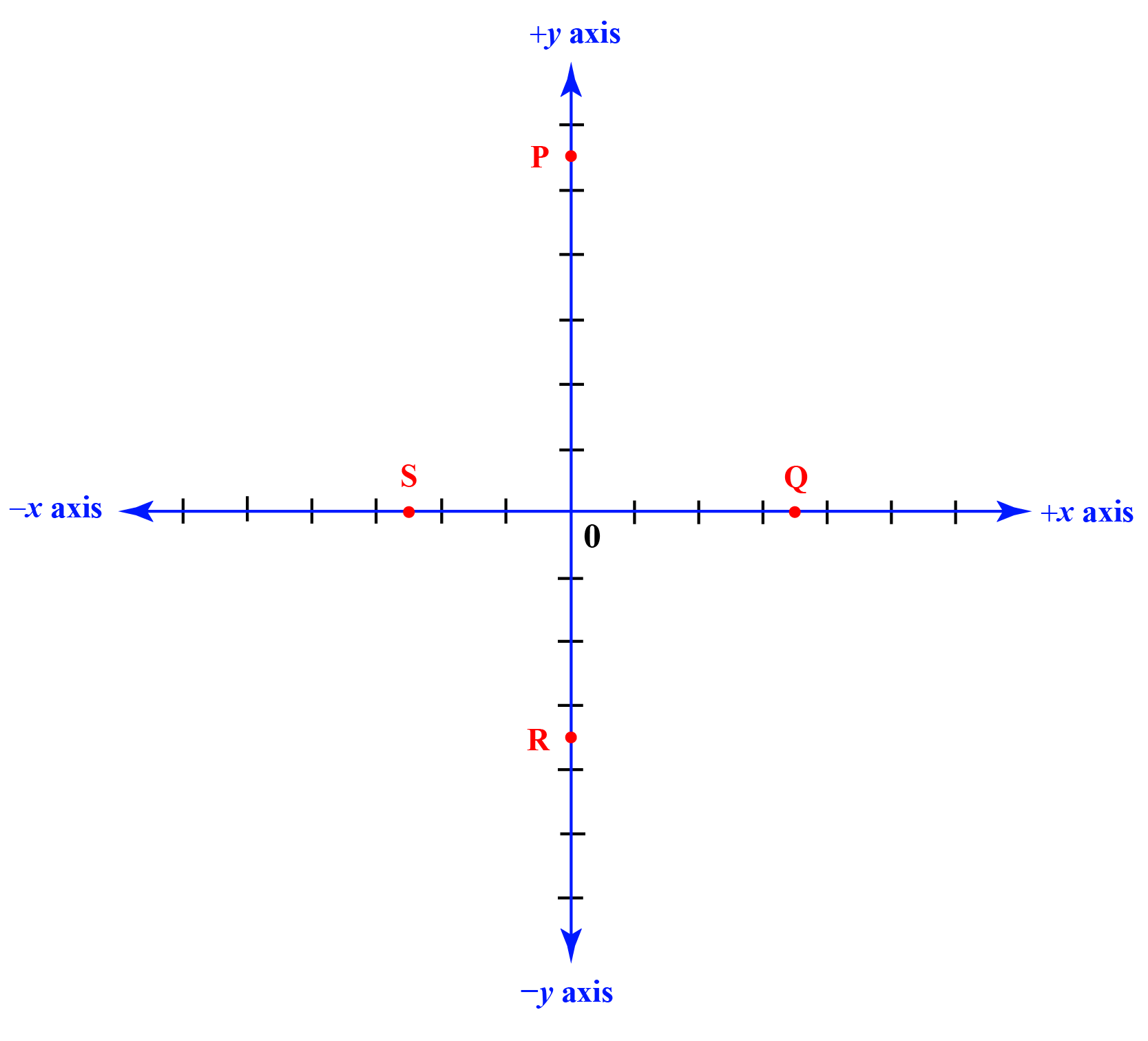Solution

If any of the points lie on the axes or if any of the coordinate value is zero, this is how we can represent those points:

 Point P lies on the positive y-axis Point Q lies on the positive x-axis Point R lies on the negative y-axis Point S lies on the negative x-axis

## Interactive Questions on Cartesian Coordinates

Here are a few activities for you to practice. Select/Type your answer and click the "Check Answer" button to see the result.

## Let's Summarize

We hope you enjoyed learning about cartesian coordinates with the simulations and practice questions. Now you will be able to easily solve problems on cartesian coordinate plane, coordinate plane quadrants, cartesian coordinate graph, and cartesian coordinate system.

At Cuemath, our team of math experts are dedicated to make learning fun for our favorite readers, the students! Through an interactive and engaging learning-teaching-learning approach, the teachers explore all angles of a topic. Be it problems, online classes, videos, or any other form of relation, it’s the logical thinking and smart learning approach that we at Cuemath believe in.

## 1. What is the difference between polar and cartesian coordinates?

When we write the cartesian coordinates of a point, we consider the distance of the point on the x-axis and y-axis.

If we consider polar coordinates of a point, then we consider the distance of the point from the origin and the angle which it forms along the x-axis.

Cartesian coordinate of a point P can be $$(x, y)$$, and the polar coordinates of the same point can be $$(r cos\theta, rsin\theta)$$, where $$r$$ is the distance of the point from the origin and $$\theta$$ is the angle formed by the line joining the origin and the point from the x- axis.

## 2. What are XY Coordinates?

x-coordinate of a point is the horizontal distance from the origin to the point and y-coordinate is the vertical distance from the origin to the point.

## 3. How to plot a point in the ccp?

To plot a point on the cartesian coordinate plane, we have to first check the x-coordinate of the point and draw a perpendicular line to x-axis on that value.

Next we will check the y-coordinate of the point and draw a perpendicular line to the y-axis on that value.

The point where these two lines intersect is the required point.

More Important Topics
Numbers
Algebra
Geometry
Measurement
Money
Data
Trigonometry
Calculus
More Important Topics
Numbers
Algebra
Geometry
Measurement
Money
Data
Trigonometry
Calculus